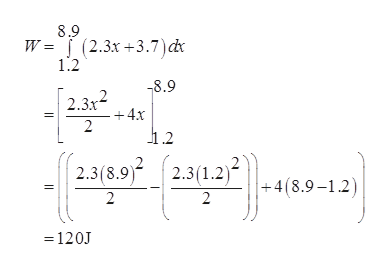# A single conservative force acts on a 5.7ˍkg particle. The equationFx=(2.3x+3.7)N describes the force, where x is in meters. As the particle moves along the x-axis from x = 1.2ˍm to x = 8.9ˍm, calculate the work done by the force on this particle, the change in the potential energy of the system, and the kineticenergy the particle has at x = 8.9ˍm if its speed is 5.4ˍm/s at x = 1.2ˍm.

Question
31 views

A single conservative force acts on a 5.7ˍkg particle. The equation
Fx=(2.3x+3.7)N describes the force, where x is in meters. As the particle moves along the x-axis from x = 1.2ˍm to x = 8.9ˍm, calculate the work done by the force on this particle, the change in the potential energy of the system, and the kinetic
energy the particle has at x = 8.9ˍm if its speed is 5.4ˍm/s at x = 1.2ˍm.

check_circle

Step 1

The formula to calculate the work done by the force in x- direction

Step 2

Substituting the valueshelp_outlineImage Transcriptionclose8.9 W (2.3x+3.7) dx 1.2 8.9 2.3x2 -+4x 2 1.2 2.3(8.9)2.3(1.2)1 +4 (8.9-1.2) 2 2 120J fullscreen
Step 3

The formula to calculate the change in potential energy of the sys...

### Want to see the full answer?

See Solution

#### Want to see this answer and more?

Solutions are written by subject experts who are available 24/7. Questions are typically answered within 1 hour.*

See Solution
*Response times may vary by subject and question.
Tagged in

### Kinematics